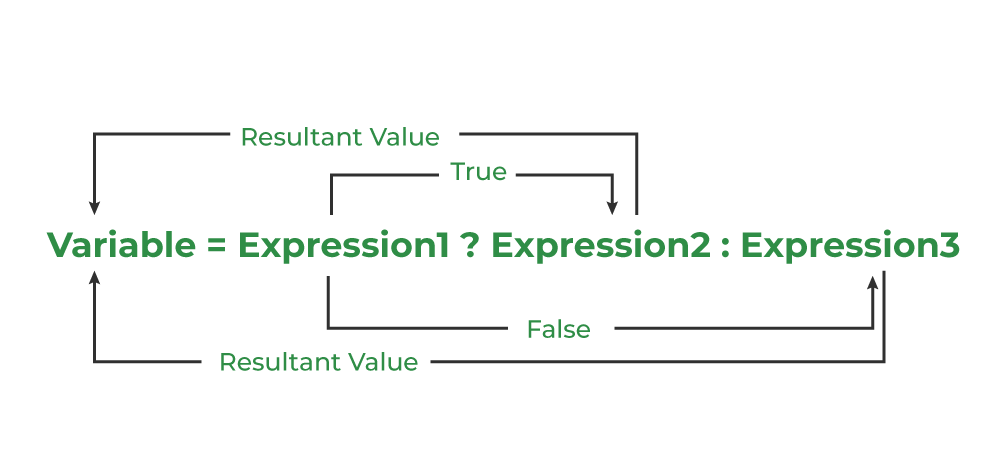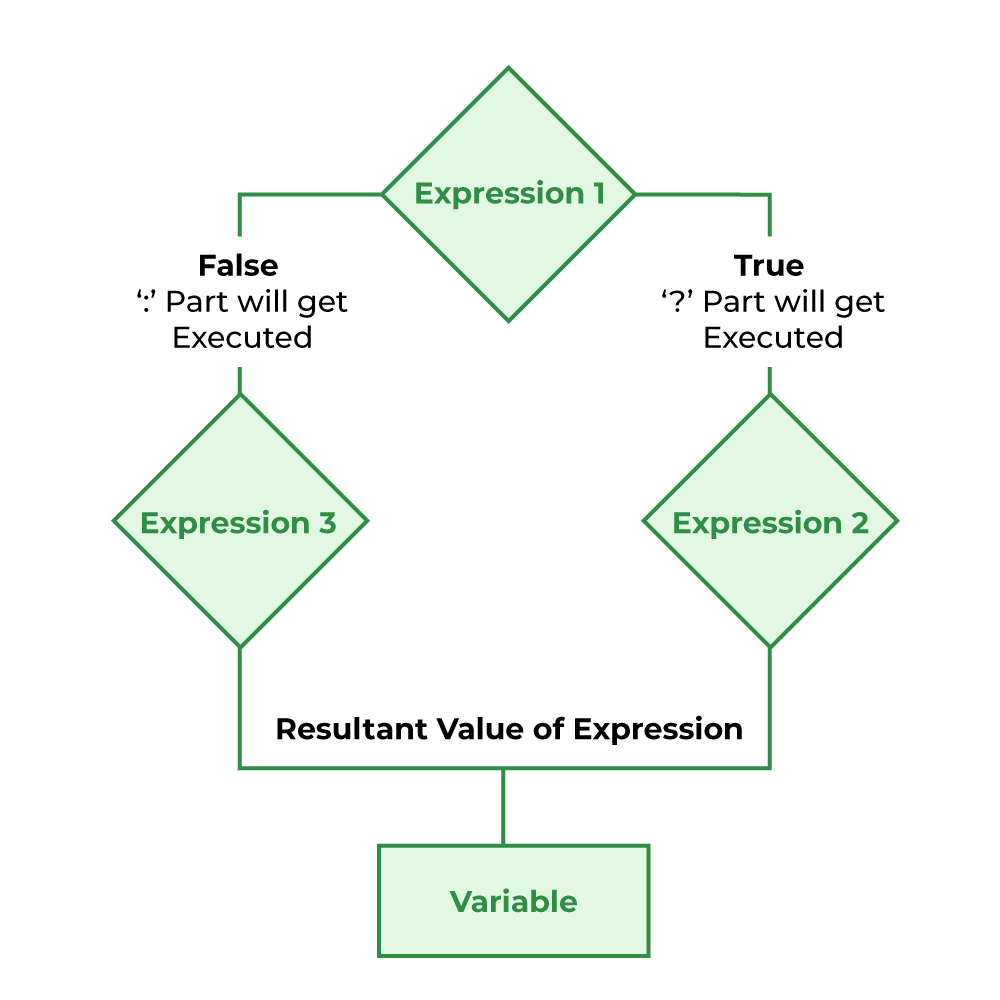Open In App
Related Articles
• Write an Interview Experience
• C Programming Language Tutorial

# Conditional or Ternary Operator (?:) in C

The conditional operator in C is kind of similar to the if-else statement as it follows the same algorithm as of if-else statement but the conditional operator takes less space and helps to write the if-else statements in the shortest way possible. It is also known as the ternary operator in C as it operates on three operands.

## Syntax of Conditional/Ternary Operator in C

The conditional operator can be in the form

`variable = Expression1 ? Expression2 : Expression3;`

Or the syntax can also be in this form

`variable = (condition) ? Expression2 : Expression3;`

Or syntax can also be in this form

`(condition) ? (variable = Expression2) : (variable = Expression3);`Conditional/Ternary Operator in C

It can be visualized into an if-else statement as:

```if(Expression1)
{
variable = Expression2;
}
else
{
variable = Expression3;
}```

Since the Conditional Operator ‘?:’ takes three operands to work, hence they are also called ternary operators.

Note: The ternary operator have third most lowest precedence, so we need to use the expressions such that we can avoid errors due to improper operator precedence management.

## Working of Conditional/Ternary Operator in C

The working of the conditional operator in C is as follows:

• Step 1: Expression1 is the condition to be evaluated.
• Step 2A: If the condition(Expression1) is True then Expression2 will be executed.
• Step 2B: If the condition(Expression1) is false then Expression3 will be executed.
• Step 3: Results will be returned.

## Flowchart of Conditional/Ternary Operator in C

To understand the working better, we can analyze the flowchart of the conditional operator given below.Flowchart of conditional/ternary operator in C

## C

 `// C program to find largest among two``// numbers using ternary operator`` ` `#include `` ` `int` `main()``{``    ``int` `m = 5, n = 4;`` ` `    ``(m > n) ? ``printf``(``"m is greater than n that is %d > %d"``,``                     ``m, n)``            ``: ``printf``(``"n is greater than m that is %d > %d"``,``                     ``n, m);`` ` `    ``return` `0;``}`

Output

`m is greater than n that is 5 > 4`

## C

 `// C program to check whether a year is leap year or not``// using ternary operator`` ` `#include `` ` `int` `main()``{``    ``int` `yr = 1900;`` ` `    ``(yr%4==0) ? (yr%100!=0? ``printf``(``"The year %d is a leap year"``,yr)``     ``: (yr%400==0 ? ``printf``(``"The year %d is a leap year"``,yr)``         ``: ``printf``(``"The year %d is not a leap year"``,yr)))``             ``: ``printf``(``"The year %d is not a leap year"``,yr);``    ``return` `0;``}`` ` `//This code is contributed by Susobhan AKhuli`

Output

`The year 1900 is not a leap year`

## Conclusion

The conditional operator or ternary operator in C is generally used when we need a short conditional code such as assigning value to a variable based on the condition. It can be used in bigger conditions but it will make the program very complex and unreadable.

## FAQs on Conditional/Ternary Operators in C

### 1. What is the ternary operator in C?

The ternary operator in C is a conditional operator that works on three operands. It works similarly to the if-else statement and executes the code based on the specified condition. It is also called conditional Operator

### 2. What is the advantage of the conditional operator?

It reduces the line of code when the condition and statements are small.